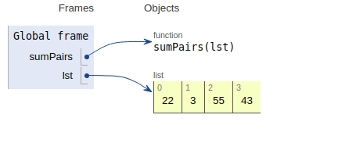# Python program to find sum of absolute difference between all pairs in a list

In this article, we will learn about the solution and approach to solve the given problem statement.

## Problem statement

Given a list input , we need to find the sum of absolute difference between all pairs in a list.

Enumerate() method adds a counter to an iterable and returns it in a form of enumerate object type.

In this method, we have a list ‘diffs’ which contains the absolute difference.

We use two loops having two variables initialized . One is to iterate through the counter and another for the list element. In every iteration, we check whether the elements are similar or not.

If not, find the absolute difference and append it to diffs list .

Finally,we find the sum of list. Since each pair will be counted twice, we divide the final sum by 2 to get the desired value and return it.

## Example

Live Demo

def sumPairs(lst):
diffs = []
for i, x in enumerate(lst):
for j, y in enumerate(lst):
if i != j:
diffs.append(abs(x-y))
return int(sum(diffs)/2)
# Driver program
lst = [22,3,55,43]
print(sumPairs(lst))

## Output

177

All the variables & functions are declared in the global scope and are shown below.## Conclusion

In this article, we learnt about the approach to find the absolute difference between all pairs in a list